# MCAT Physical : Density

## Example Questions

← Previous 1

### Example Question #5 : Submersion

What is the density of a log that is 25cm long, has a cross sectional area of  5cm2, and weighs 100g?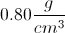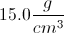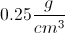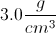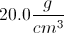Explanation:

The density of an object is equal to mass over volume.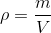Knowing the length and the cross sectional area of the log, we can find its volume.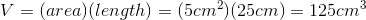Plugging in volume and mass into the equation will enable us to find density.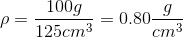### Example Question #1 : Submersion

Solid A has a volume of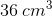and a density of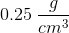. Solid B is cube with sides of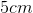and has a density of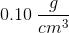.

What is the difference in mass between the two solids?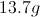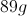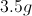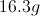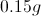Explanation:

The formula for density is: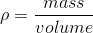In the question, we are given the densities of both solids and a means to find their volumes. Using these values, we will be able to determine the mass of each solid.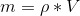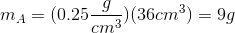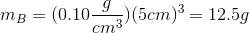Now that we know both masses, we can find the difference.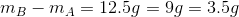### Example Question #7 : Submersion

A researcher performs an elemental analysis on a compound. He finds that the compound is made up of only carbon, hydrogen, and oxygen atoms. He isolates a pure sample of the compound and finds that this sample contains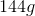of carbon,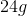of hydrogen, and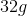of oxygen. The researcher wants to perform further analysis on this compound the next day. Before leaving the lab the researcher creates three stock solutions of varying concentrations of this compound: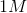(solution A),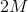(solution B), and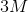(solution C). He stores these solutions overnight at a temperature of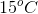.

Molecular weight of this compound =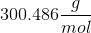Astock solution, solution X, kept at room temperature (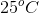) will have __________ compared to solution A.

a greater density

the same density

a lower density

a lower density

Explanation:

Solution A and solution X have the same concentration, therefore, we are only concerned with temperature differences between the two solutions. Density is dependent on temperature: as temperature increases density decreases. Recall the definition of density: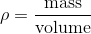Increasing the temperature will slightly increase the volume of the solution and, subsequently, decrease density. The temperature has no effect on mass. The solution at the higher temperature (solution X) will have a lower density.

### Example Question #8 : Submersion

Various sample sizes of a pure, unknown substance are measured for mass (in grams) and volume (in mL). The results are plotted on a graph with grams of the y-axis and milliliters on the x-axis. Which of the following results most likely indicates experimental error?

A linear slope

A negative slope

A slope very close to zero

A slope greater than one

A negative slope

Explanation:

Plotted in this way, the slope of the line is equal to the density of the substance.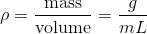We would expect the line to be linear and positive. A slope close to zero could indicate that the data was not graphed optimally or that the density is extremely small, and a density greater than one is possible (anything that sinks in water has a density greater than one). A negative density would indicate that greater masses of this substance have smaller volumes, which, for a pure substance, does not make sense.

### Example Question #2 : Submersion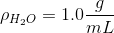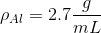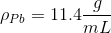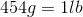Which of these samples, if any, has a volume greater than three liters?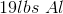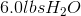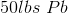Both the aluminum and water samplesNone of these has a volume greater than three litersExplanation:

Solving this question requires that we use the given densities to convert mass to volume.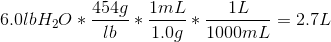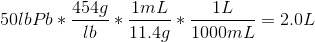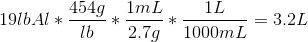We see that only the aluminum sample has a volume over three liters.

### Example Question #10 : Submersion

What is the specific gravity of a boat that has a mass of 6000kg and a volume of 10m3?

6

10

0.6

100

1

0.6

Explanation:

Specific gravity is the density of the substance over the density of water.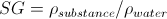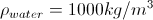Density is given by mass over unit volume.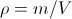A boat with a mass of 6000kg and a volume of 10m3 will have a density of 600 kg/m3.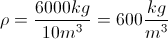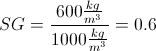### Example Question #11 : Submersion

A 200-gram object is placed into a bucket of water and floats so that its top edge is just below the surface of the water. Which best describes the density of this object?

Density of water is 1000kg/m3.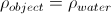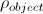varies depending on the depth of the object below the surfacemay be different depending on the volume of water in the bucket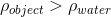Explanation:

If the object is at rest, the net force on it must equal 0. At any point in the liquid, the total downwards force is described as the difference between the gravitational force and bouyant force: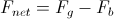. When the object is not accelerating, this reduces to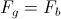.

Plugging in the equations for these forces respectively yields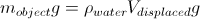.

Since mass is the product of density and volume,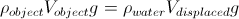.

Note that in this case the object's volume equals the volume of liquid displaced, since the object is completely submerged. So, the volumes on either side cancel, as does gravity, leaving just.

### Example Question #12 : Submersion

An ice cube is placed into a glass containing an unknown liquid. If the ice cube sinks to the bottom, which of the following conclusions could be made?

The liquid has a density greater than that of water

The liquid has a density less than that of water

The density of the liquid is equal to the density of water

No conclusions can be made unless the temperature of the liquid is known

No conclusions can be made unless the mass of the ice cube is known

The liquid has a density less than that of water

Explanation:

The density of ice is less than that of water (density of ice = 0.92 g/cm3, density of water = 1 g/cm3). Ice will only sink in liquids that are less dense than 0.92 g/cm3. Since ice will only sink in liquids that are less dense than water, the unknown liquid must have a lower density than that of water.

### Example Question #1 : Density

Phase diagrams are used to depict changes in the properties of a solution at different temperatures and pressures. Below is a phase diagram of a polar solution.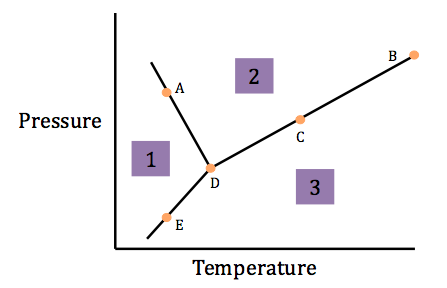The density of the solution in section 1 is __________ the density of the solution in section 2.

greater than

less than

equal to

less than

Explanation:

The slope of the solid/liquid phase transition line can predict the comparative density of the solution in each section. A negative slope indicates that the solid phase is less dense than the liquid phase. A positive slope indicates that the solid is more dense. In the above phase diagram, the slope is negative, indicating that the solid is less dense than the liquid.

### Example Question #1 : Density

An object is at equilibrium when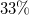of the total volume is submerged in gasoline. Find the density of the object.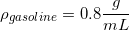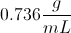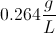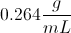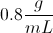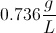Explanation:

To solve, we can equate the volume of the gasoline displaced to the volume of the portion of the object that is submerged.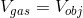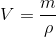We know thatof the object is submerged, thusof the object's total volume will equal the volume of water displaced.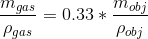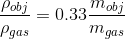The displaced masses are equal.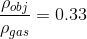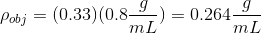← Previous 1